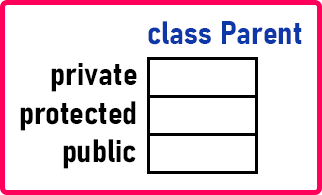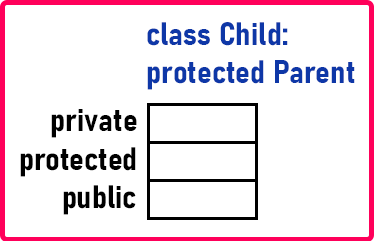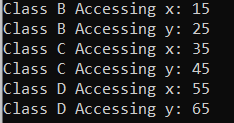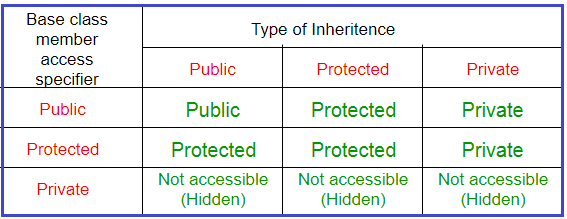# Modes of Inheritance in C++

## Modes of Inheritance in C++ with Examples

In this article, I am going to discuss Modes of inheritance in C++ with Examples. Please read our previous article where we discussed Types of Inheritance in C++ with Examples.

##### Modes of Inheritance in C++:

Let us see how we can derive a class from the base class. There is more than one way of deriving. For a better understanding, please have a look at the below code.

```class Base
{
private:
int a;
protected:
int b;
public:
int c;
};
class Derived:Base
{
public:
fundDerived ()
{
a = 1;
b = 2;
c = 3;
}
};```

There are three modes of inheritance that is publicly, privately, and protected. If we are not writing any access specifiers then by default it becomes private. So, the same thing I will show you with the help of a diagram.Suppose we have a class Parent class which have private, protected, and public data members. Then we have another class that is Child class. The Child class is also having private, protected, and public members. Let’s say the Child Class is publicly inheriting from the Parent class as shown in the below image.As the Child Class is inheriting publicly, everything is available here. But what are accessible? Only protected and public members are accessible.

###### When the protected or public members of the base class come into the derived class then what do they become?

See when we are inheriting publicly, the protected members of the base class will become protected in the derived class and public members of the base class will become public in the derived class. So, it means when we create an object of Child class, we can access only public members. Suppose we have another class that is Grandchild class which is publicly inheriting from the Child class as follows.Here Grandchild class has also private, protected, and public members. So whatever protected members present in the Child class will be accessible protected in the Grandchild class and whatever public members are there in the Child class will be accessible in public in the Grandchild class. Now let’s see the difference. If we make Child class protected inheriting from Parent class as follows.Then the protected members of the Parent will become protected in the Child class as well as the public members of the Parent will also become protected in the Child class. Now one more method if we inherit the Base class privately from the Parent class as follows.Then the protected members and public members of the Parent class will become private in the Child class. Now when the GrandChild class inherits from the Child class then is it able to access private members of the Child class? No. The GrandChild class cannot access the members of the Parent class.

##### Real-Time Example to Understand Modes of Inheritance:

Suppose I have a car company X and this company has its own design. Now another car company called Y has borrowed that design from my company X. So, whatever is there in the X design, the same thing will be available in Y design. But Y design cannot access everything. Y can access the protected and public parts. It cannot access the private part.

Now further Y company is giving that design to some other company Z. The Company Y can provide that design to other company Z because Y has paid to X company. So, Y can sell that design as it has permission to sell that design further. Now what you want to access by the 3rd company that is ‘Z’.

1. If Y has taken publically from X, then Z company can access everything.
2. If Y has taken privately from X, then Z cannot modify anything, it can just use that design. The design will be available to Z but it cannot modify anything. It can add extensions but the existing things cannot be modified.
3. Now if Y has taken protected from X then Z can modify some things but further, it is restricted. So, X gives some access to Y then Y gives access to Z.

So, we are just passing down the access to some other company. Now how much it can access that is the point here. You can restrict access down the hierarchy of classes. This is not available in other languages. It’s not in Java and C#. You cannot inherit publicly or privately. There is only one type of inheritance in Java and C#.

##### Mode of Inheritances in C++:

A class can be inherited in flowing ways

1. Public – All members of the base will have the same accessibility in the derived class. That means if we derive a subclass from a public base class. Then the public member of the base class will become public in the derived class and protected members of the base class will become protected in the derived class.
2. Protected – All members of the base will become protected in the derived class. That means if we derive a subclass from a Protected base class. Then both public members and protected members of the base class will become protected in the derived class.
3. Private – All members of the base will become private in the derived class. That means if we derive a subclass from a Private base class. Then both public members and protected members of the base class will become Private in the derived class.

Note: The private members of the base class cannot be directly accessed in the derived class, while protected members can be directly accessed.

So, these are the modes of inheritance. Now let us write the program for this one.

##### Example to Understand Different Access Modes of Inheritance in C++:
```#include <iostream>
using namespace std;
class A
{
private:
int z;

protected:
int y;

public:
int x;

void funA()
{
x = 10;
y = 5;
z = 15;
}
};

class B:public A
{
// x is public
// y is protected
// z is not accessible from B
public:
void funB()
{
x = 15;
y = 25;
//z=25; //Error
}
void DispalyB()
{
cout << "Class B Accessing x: " << x << endl;
cout << "Class B Accessing y: " << y << endl;
// We cannot access z as it is private in class A
//cout << "Class B Accessing z: " << z;
}
};

class C:protected A
{
// x is protected
// y is protected
// z is not accessible from C
public:
void funC()
{
x = 35;
y = 45;
//z=45; //Error as it is private in A
}
void DispalyC()
{
cout << "Class C Accessing x: " << x << endl;
cout << "Class C Accessing y: " << y << endl;
// We cannot access z as it is private in class A
//cout << "Class C Accessing z: " << z;
}
};

class D:private A		// 'private' is default for classes
{
// x is private
// y is private
// z is not accessible from D
public:
void funD()
{
x = 55;
y = 65;
//z=45; //Error
}
void DispalyD()
{
cout << "Class D Accessing x: " << x << endl;
cout << "Class D Accessing y: " << y << endl;
// We cannot access z as it is private in class A
//cout << "Class D Accessing z: " << z;
}
};

int main()
{
B b;
//Only public members are accessible from outside the class
b.x = 10;
//Cannot access the protected and private data members from outside the class
//b.y = 5;
//b.z = 20;
b.funB();
b.DispalyB();

C c;
//Cannot access the protected and private data members from outside the class
//c.x = 10;
//c.y = 5;
//c.z = 20;
c.funC();
c.DispalyC();

D d;
//Cannot access the private data members
//d.x = 10;
//d.y = 5;
//d.z = 20;
d.funD();
d.DispalyD();
}
```
###### Output:The below table summarizes the three modes of inheritances and shows the access specifier of the members of the base class in the subclass when derived in public, protected, and private modes: